# SQL Server 2019 & Java Null Handling: Take Two

Posted by nielsb on Saturday, October 26, 2019

You who read my blog know that during the last year, (or so), I have been writing about SQL Server 2019 and the ability to call into Java code from SQL Server:

It has been a fascinating “journey”, since SQL Server 2019 is still in preview, and there have been changes in how you call Java code along the way. In this post, we look at some relatively recent changes to how we handle null values in datasets.

## Java Code in SQL Refresh

If you have not done any Java code in SQL Server, or at least not recently, here are a couple of posts which introduces you to Java in SQL Server:

## Demo Code

#### Data

In today’s post, we use some data from the database. The code to insert the data is the same we used in a previous post: SQL Server 2019 Extensibility Framework & Java - Null Values:

 `````` 1 2 3 4 5 6 7 8 9 10 11 12 13 14 15 16 17 18 19 20 21 22 23 24 25 26 27 28 29 30 31 32 33 34 35 36 37 38 39 `````` ``````USE master; GO SET NOCOUNT ON; GO DROP DATABASE IF EXISTS JavaNullDB; GO CREATE DATABASE JavaNullDB; GO USE JavaNullDB; GO DROP TABLE IF EXISTS dbo.tb_NullRand10 CREATE TABLE dbo.tb_NullRand10(RowID int identity primary key, x int, y int, col1 nvarchar(50)); GO INSERT INTO dbo.tb_NullRand10(x, y, col1) SELECT TOP(10) CAST(ABS(CHECKSUM(NEWID())) % 14 AS int) , CAST(ABS(CHECKSUM(NEWID())) % 20 AS int) , N'Hello ' + CAST(CAST(ABS(CHECKSUM(NEWID())) % 25 AS int) AS nvarchar(50)) FROM sys.objects o1 CROSS JOIN sys.objects o2 CROSS JOIN sys.objects o3 CROSS JOIN sys.objects o4; GO UPDATE dbo.tb_NullRand10 SET y = NULL WHERE RowId = 3 UPDATE dbo.tb_NullRand10 SET Col1 = NULL WHERE RowId = 5 UPDATE dbo.tb_NullRand10 SET x = NULL WHERE RowId = 6 UPDATE dbo.tb_NullRand10 SET y = NULL WHERE RowId = 8 UPDATE dbo.tb_NullRand10 SET col1 = NULL WHERE RowId = 9 GO ``````

Code Snippet 1: Create Database Objects

We see from *Code Snippet 1 * how we:

• Create a database: `JavaNullDB`.
• Create a table: `dbo.tb_NullRand10`.
• Insert some data into the table.

The data we insert is entirely random, but it gives us something to “play around” with.

Let us see what the data looks like, by executing: `SELECT RowID, x, y FROM dbo.tb_NullRand10;`: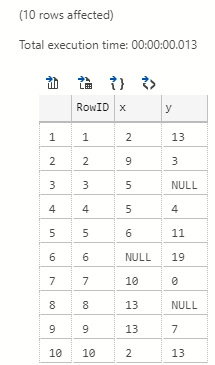Figure 1: T-SQL Result

As we see in Figure 1, we get back 10 rows, and rows 3, 6, and 8 contains null values.

#### Enable Java in the Database

To use Java in the database, you need to do a couple of more things:

Now, when we have a database with some data and Java enabled we, can start.

## Null Values

In the null values post mentioned above, I mentioned that there are differences between SQL Server and Java in how they handle null. So, when we call into Java from SQL Server, we may want to treat null values the same way as we do in SQL Server.

I wrote about this in the SQL Server 2019 Extensibility Framework & Java - Null Values post mentioned above. However, that post was written before SQL Server 2019 CTP 2.5. In CTP 2.5 Microsoft introduced the Java SDK, and certain things changed. Amongst the things that changed is the way we handle nulls when we receive datasets from SQL Server in our Java code.

Let us see how null handling works now post CTP 2.5. We use similar code to what we saw in the null post above:

 `````` 1 2 3 4 5 6 7 8 9 10 11 12 13 14 15 16 17 18 19 `````` ``````public PrimitiveDataset execute(PrimitiveDataset input, LinkedHashMap params) { int[] inputCol1 = input.getIntColumn(0); int[] inputCol2 = input.getIntColumn(1); int[] inputCol3 = input.getIntColumn(2); PrimitiveDataset output = new PrimitiveDataset(); output.addColumnMetadata(0, "RowID", Types.INTEGER, 0, 0); output.addColumnMetadata(1, "x", Types.INTEGER, 0, 0); output.addColumnMetadata(2, "y", Types.INTEGER, 0, 0); output.addIntColumn(0, inputCol1, null); output.addIntColumn(1, inputCol2, null); output.addIntColumn(2, inputCol3, null); return output; } ``````

Code Snippet 2: Java Input & Output Data

The code in Code Snippet 3 “echoes” back what it receives as input dataset and we see how we:

• Load three arrays with the three columns in the dataset.
• Create a new `PrimitiveDataset` to use as the return type.
• Set metadata for the return dataset.
• Assign the columns for the return dataset.
• Return the dataset.

NOTE: If you wonder about why the method is named `execute` and what the `PrmitiveDataset` is; the post here explains it.

After we have compiled and packaged the code into a `.jar` file we can deploy:

 ``````1 2 3 `````` ``````CREATE EXTERNAL LIBRARY SqlNullLib FROM (CONTENT = 'W:\sql-null-1.0.jar') WITH (LANGUAGE = 'Java'); ``````

Code Snippet 3: Deploy Java Code

As we see in Code Snippet 3 I named my `.jar` file `sql-null-1.0.jar` and I deployed it as an external library: `SqlNullLib`. Since I deploy to a local SQL Server instance, I can use a file location for my `.jar`.

NOTE: The Java & External Libraries post mentioned above goes into details about external libraries.

The code to execute looks something like so:

 ``````1 2 3 4 5 `````` ``````EXEC sp_execute_external_script @language = N'Java' , @script = N'sql.NullValues' , @input_data_1 = N'SELECT RowID, x, y FROM dbo.tb_NullRand10' WITH RESULT SETS((RowID int, x int, y int)) ``````

Code Snippet 4: Execute Code

We see in Code Snippet 4 how we call into the `NullValues` class in the `sql` package and how we use the same `SELECT` statement that generated the resultset we saw in Figure 1. When we execute the code, we see: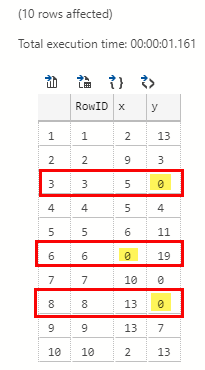Figure 2: Java Code Result

Compare the result we see in Figure 2 with the result in Figure 1, and we see the difference in the outlined rows, (3, 6, 8), and the highlighted columns, In Figure 1 the columns are `NULL`, whereas in Figure 2 they are `0`. So why are the columns `0`?

Well, as we said in the null post, this is because the Java language C++ extension converts the null values to the default value for the data type in question.

NOTE: The Java language C++ extension is the bridge between SQL Server and your Java code. The SQL Server 2019 Extensibility Framework & External Languages post covers it in some detail.

The question is, why do we care that a null value comes across as zero, at least we do not get a null exception? Let us take a look at the following Java code:

 `````` 1 2 3 4 5 6 7 8 9 10 11 12 13 14 15 16 17 18 19 `````` ``````public PrimitiveDataset execute(PrimitiveDataset input, LinkedHashMap params) { int[] inputCol3 = input.getIntColumn(2); int numRows = inputCol3.length; int sum = 0; for(int x = 0; x < numRows; x++) { sum += inputCol3[x]; } double avg = (double)sum / numRows; System.out.printf("Average value of y is: %f", avg); return null; } ``````

Code Snippet 5: Average Value

The code in Code Snippet 5 expects the same input data as we generated in Code Snippet 4, and it calculates the average value of the `y` column of that dataset. When we execute the code in Code Snippet 4, after having compiled, packaged and deployed, (comment out `WITH RESULT SETS`), we see the result as so:Figure 3: Result of Average Calculation

The result in Figure 3 looks OK, so let us see what it looks like if we run a similar query in SQL Server:

 ``````1 2 `````` ``````SELECT CAST(AVG(CAST(y AS DECIMAL(4,2)))AS DECIMAL(4,2)) FROM dbo.tb_NullRand10; ``````

Code Snippet 6: T-SQL Average Calculation

The result of the query in Code Snippet 6 looks like so: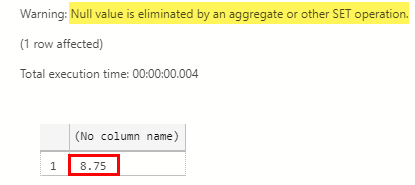Figure 4: Result of T-SQL Average Calculation

We see in Figure 4 how the result of the average calculation, (outlined in red), differs from the Java calculation. The question is why this is; it was the same data in both calculations? Well, was it; we see in Figure 4 the highlighted part at the top: “Null value is eliminated …”. So what happens is that for certain operations SQL Server eliminates null values, as SQL Server treats nulls as unknown.

As the Java language C++ extension converts nulls, we need to handle it in our Java code.

#### Input Nulls

In the previous null post we saw that, when we want to handle null input, we use a required class level variable `inputNullMap`, which the Java language extension populates “automagically”. However, after the introduction of the Java language SDK, this variable is not required any more. Even if you declared it, the Java language extension does not populate it.

So how do we then figure out whether a column has a null value? Well, since the Java language extension passes data into the `execute` method via the `PrimitiveDataSet` class, let us have a look at the base class for `PrimitiveDataSet`: `AbstractSqlServerExtensionDataset`:

 `````` 1 2 3 4 5 6 7 8 9 10 11 12 13 14 15 16 17 `````` ``````public class AbstractSqlServerExtensionDataset { /** * Column metadata interfaces */ public void addColumnMetadata(int columnId, String columnName, int columnType, int precision, int scale) { throw new UnsupportedOperationException("addColumnMetadata is not implemented"); } ... public boolean[] getColumnNullMap(int columnId) { throw new UnsupportedOperationException("getColumnNullMap is not implemented"); } ... } ``````

Code Snippet 7: AbstractSqlServerExtensionDataset

We see in Code Snippet 7 how the `AbstractSqlServerExtensionDataset` has a section for metadata, and in that section is a method: `getColumnNullMap`. The method takes an integer as an input parameter, and it returns an array of type `boolean`.

NOTE: The Java SDK is open source, and you find it here.

This is what happens when the Java language C++ extension populates the dataset which is used as an input parameter:

• The extension creates a `boolean` array for each non-nullable Java datatype columns.
• The extension loops each row for each column in the dataset.
• Where there is a null value, for a primitive data type, the extension assigns the default value of the data type to that column.
• When the extension comes across a null value in a non-nullable Java data type column, it sets the boolean array value to `true` in the column array.

With this in mind we can change the code in Code Snippet 5 to handle null values, or rather handle values in the dataset that originates from a SQL Server null value:

 `````` 1 2 3 4 5 6 7 8 9 10 11 12 13 14 15 16 17 18 19 20 21 22 23 24 `````` ``````public PrimitiveDataset execute(PrimitiveDataset input, LinkedHashMap params) { int[] inputCol3 = input.getIntColumn(2); int numRows = inputCol3.length; boolean[] nullMap = input.getColumnNullMap(2); int nonNull= 0; int sum = 0; for(int x = 0; x < numRows; x++) { if(!nullMap[x]) { nonNull ++; sum += inputCol3[x]; } } double avg = (double)sum / nonNull; System.out.printf("Average value of y is: %f", avg); return null; } ``````

Code Snippet 8: Null Handling

So, in Code Snippet 8 we see how we:

• Get the column we want to create the average over.
• Use `getColumnNullMap` to retrieve the null map for the column we use for the calculation.
• In the `for` loop check whether the column value is null or not. If it is not null, we include the value and increase the row count.
• Finally do the average calculation.

The result when executing the code in Code Snippet 4 against our new code looks like so: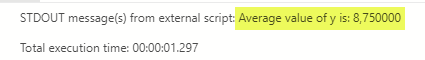Figure 5: New Java Average Calculation

We see in Figure 5 how our Java calculation now gives the same result as the T-SQL calculation. Awesome!

#### Output Nulls

We have now seen how to use `getColumnNullMap` to distinguish input values that come in as `NULL` from SQL Server, which the Java language C++ extension converts to the default value for the Java data type.

What about if we need to return null values to SQL Server in a return dataset, but the Java data type is non-nullable? I.e. we receive data in the input dataset, and some column values for a non-nullable Java type are null when passed in from SQL Server. If we wanted to, for example, add the column to another column, the sum should be `NULL` if we were to handle it the same way as SQL Server does.

So how do we indicate to SQL Server that a column value is null, even though it has a value in Java? Let us go back to Code Snippet 2 where we discussed how to return data to SQL Server from Java code. After “newing” up an instance of `PrimitiveDataset`, we defined the metadata for the columns via the `addColumnMetadata` method. We then added the row arrays for the columns through the `add*Type*Column`, (in our case it was `addIntColumn`), and it is in that method the “secret” to null values lies. Let us go back to `AbstractSqlServerExtensionDataset` and look at the signature for `addIntColumn`:

 `````` 1 2 3 4 5 6 7 8 9 10 `````` ``````public class AbstractSqlServerExtensionDataset { /** * Adding column interfaces */ public void addIntColumn(int columnId, int[] rows, boolean[] nullMap) { throw new UnsupportedOperationException(...); } } ``````

Code Snippet 9: Add Column Method

Look in Code Snippet 9 at the third parameter in the add method. See how it takes a `boolean` array, and how the name “gives it away”: `nullMap`. If we look at other methods for non-nullable Java types, we see that all of them have this parameter, whereas add methods for types that are nullable do not have it.

So for non-nullable types, we define `boolean` arrays, and in those arrays, we indicate what row value(s) is `null`. Let us see an example:

 `````` 1 2 3 4 5 6 7 8 9 10 11 12 13 14 15 16 17 18 19 20 21 22 23 24 25 26 27 28 29 30 31 32 33 34 35 36 37 38 39 40 41 `````` ``````public PrimitiveDataset execute(PrimitiveDataset input, LinkedHashMap params) { PrimitiveDataset output = new PrimitiveDataset(); int numRows = 5; output.addColumnMetadata(0, "RowID", Types.INTEGER, 0, 0); output.addColumnMetadata(1, "IntCol", Types.INTEGER, 0, 0); output.addColumnMetadata(2, "StringCol", Types.NVARCHAR, 256, 0); int[] rowIdRows = new int[numRows]; int[] intColRows = new int[numRows]; String[] stringColRows = new String[numRows]; boolean[] intColNullMap = new boolean[numRows]; for(int x = 0; x < numRows; x++){ rowIdRows[x] = x+1; if(x % 2 ==0) { intColRows[x] = 0; intColNullMap[x] = true; } else { intColRows[x] = x + 2; intColNullMap[x] = false; } if(x % 3 ==0) { stringColRows[x] = null; } else { stringColRows[x] = "Hello number: " + (x + 1); } } output.addIntColumn(0, rowIdRows, null); output.addIntColumn(1, intColRows, intColNullMap); output.addStringColumn(2, stringColRows); return output; } ``````

Code Snippet 10: Return Dataset

What we see in Code Snippet 10 is a somewhat contrived example where we return a dataset in which we want certain column values to be `NULL` in SQL Server. We see how we:

• Create metadata for the dataset.
• Create arrays for the individual rows.
• Create a null map for one of the integer columns.
• In the `for` loop add values to the arrays, and based on some modulus operations emulate that some values are null.
• Add the arrays to the columns, and for the second integer column, we also add the null map.
• Finally return the dataset.

A couple of things to notice in the code in Code Snippet 10:

• A null-map is not required for a non-nullable data type columns if the values are not null.
• We do not need a null-map for nullable data type columns.

To see that our code works we use following code:

 ``````1 2 3 4 `````` ``````EXEC sp_execute_external_script @language = N'Java' , @script = N'sql.NullValues' WITH RESULT SETS((RowID int, IntCol int, StringCol nvarchar(256))) ``````

Code Snippet 11: Execute T-SQL

The result we get when executing the code in Code Snippet 11, looks like so: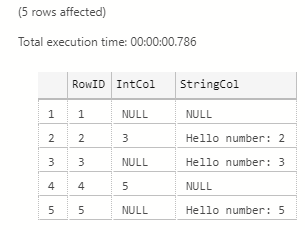Figure 6: Return Dataset with Null Values

When we look at Figure 6 we see that our code worked, and how the Java C++ language extension converted the `0` values in the integer column to `NULL` based on the null map.

## Summary

In this post, we discussed how to handle null values in datasets passed into our Java code from SQL Server, and from our Java code back to SQL Server. I wrote about null handling in a previous post, but since that post, Microsoft introduced the Java language SDK for SQL Server, and null handling has changed.

We care about null values because, in SQL Server, all data types are nullable, whereas, in Java, that is not the case. In Java, like in .NET, primitive types, (`int`, etc.), cannot be null. The Java C++ language extension handles the mismatch between nullable in SQL Server and non-nullable in Java, whereby it converts null values in data from SQL Server to the data type’s default value for Java. Going the other way, from Java to SQL Server, the C++ language extension converts the values supposed to be null to actual null values.

The way we handle null values after the introduction of the Java language SDK is that we:

• For input data, and each non-nullable column, we call `getColumnNullMap` and pass in the columns ordinal position. We then handle the values where the null map indicates a null value.
• For output data we create `boolean` arrays for the columns which should contain null values in SQL Server. We pass the array in as a parameter to the `add*TypeName*Column` method.

## ~ Finally

If you have comments, questions etc., please comment on this post or ping me.